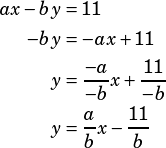Daily PWN 003:

y= 3x+4

ax-by= 11 Find (b/a). The system has no solutions.

Is ax-by=11 in standard form of a line? If so, doesn’t the formula -(A/B) equal the slope? A has to positive, so A=3 and B= -1. So, -(3/-1)= 3… so B/A= -1/3. The actual answer is 1/3. Can you explain to me what I did wrong? Thanks!

You’re losing a negative sign when you calculate the slope of the second equation. To see why, manipulate it a bit to get it into slope-intercept form:When a linear system has no solutions, you know you’re dealing with parallel lines. Once you have both equations in slope-intercept form, it’s easy to see thatmust equal 3 to make the lines parallel. If that’s true, then the reciprocal.